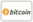Borítókép a Metrics in Fuzzy Graph - hoz
Könyv címe:

# Metrics in Fuzzy Graph

LAP LAMBERT Academic Publishing (21.01.2015 )Érvényes utalvány
ISBN-13:

### 978-3-659-67937-7

ISBN-10:
3659679372
EAN:
9783659679377
Könyv nyelve:
angol
Ismertető:
In this book 50 metrics in weighted graph and 53 metrics in fuzzy graph are introduced.Using 3 metrics the geodesics, convex hull,etc are introduced.The researchers can use these metrics to develop self centred fuzzy graphs and weighted graphs to simulate the transportation problems and communication networks.This book will be very useful to the researchers in graph theory, fuzzy mathematics ,and communication networks.
Weboldal:
https://www.lap-publishing.com/
Szerezte:
Oldalszám:
72
21.01.2015
Raktárkészlet:
Raktáron
Kategória:
Matematika
Ár:
39,90 €
Kulcsszavak:
graph theory, Fuzzy Graphs, Fuzzy Mathematica### Kategóriák